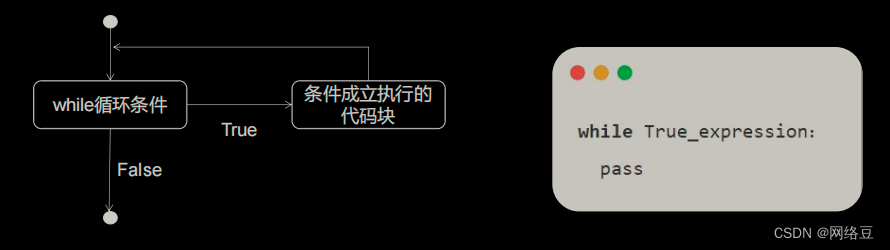# Python编程 while循环

+关注继续查看

## 一.循环控制

### 2.while循环表达式### 3.while循环表达式

# while True:
#     print("hello everyone")

#初始化i，i相当于计数器，记录循环的次数
i = 1
while i <= 5:
print("hello")
i = i + 1

#1+2+3+4+5+......+100
n = 1
num_sum = 0

#循环不可逆，只能一直往下循环
while n <= 100:
num_sum = num_sum + n # 0+1+2.....+100
n = n + 1

print(num_sum)   #5050

### 4.break：

#1+2+3+4+5+......+100
n = 1
num_sum = 0

#循环不可逆，只能一直往下循环
while n <= 100:
num_sum = num_sum + n # 0+1+2.....+100
print(n)
if n == 8:
break
#break 退出循环。注意：是循环的语句，不是if的语句
n = n+1
print(num_sum)   #5050

### 5.continue：

continue 语句用于 跳过 当前循环体 剩余部分的代码，直接 开始下一轮循环。它 不会退出和终止

#1+2+3+4+5+......+100
n = 1
num_sum = 0
#循环不可逆，只能一直往下循环
while n <= 100:
num_sum = num_sum + n # 0+1+2.....+100

n = n + 1  # 注意：根本不执行n+1这个代码，所以意味着n一直为8
if n == 8:

continue
#continue:退出当前循环，进入下次循环
print(n)

print(num_sum)   #5050

### 6.else结构

while 的 else 从句

while 循环还可以增加一个 else 从句。当 while 循环 正常执行 完毕，会执行 else

else 与 while 平级的缩进方式

while n <= 100:
num_sum = num_sum + n   # 0+1+2+3+...+100
n = n + 1
if n == 8:
# continue
# break
continue
print(n)
else:
# 当循环是正常结束的时候，执行else
# break是强制结束循环体，所以不会执行else里面内容
print("我是else")

### 7.while循环嵌套使用

if 判断可以嵌套，while 当然也可以嵌套。但是建议大家不要嵌套 3 层以上，那样的

*

**

***

#*
#**
#***
#****
#*****

# row = 1
# while row <= 4:
#     print("*"* row)
#     row += 1

#嵌套实现
row = 1
while row <= 4:
starts = 1
while starts <=row:
print("*",end='')
# print(row,starts)
starts += 1
print("")
row += 1|
1天前
|

python之for循环和while循环的使用教程，小白也能学会的python之路
python之for循环和while循环的使用教程，小白也能学会的python之路
12 0
|
13天前
|

Python学习手册--第四部分(用户输入和while循环)
Python学习手册--第四部分(用户输入和while循环)
17 0
|
23天前
|
Python
Python循环结构while\for-in
Python循环结构while\for-in
9 0
|
1月前
|

Python学习笔记丨条件与循环流程控制，if、while、for基础知识总结与易错点分享
Python学习笔记丨条件与循环流程控制，if、while、for基础知识总结与易错点分享
23 0
|
1月前
|

Python控制语句，if语句、for语句和while语句
Python控制语句，if语句、for语句和while语句
24 1
|
2月前
|

Python入门教程：掌握for循环、while循环、字符串操作、文件读写与异常处理等基础知识（下）
Python入门教程：掌握for循环、while循环、字符串操作、文件读写与异常处理等基础知识（下）
37 0
|
2月前
|

Python入门教程：掌握for循环、while循环、字符串操作、文件读写与异常处理等基础知识（上）
Python入门教程：掌握for循环、while循环、字符串操作、文件读写与异常处理等基础知识
36 0
|
3月前
|

15.从入门到精通:Python循环语句 while 循环 无限循环 while 循环使用 else 语句 简单语句组 for 语句 for...else
15.从入门到精通:Python循环语句 while 循环 无限循环 while 循环使用 else 语句 简单语句组 for 语句 for...else
33 0
|
3月前
|
Python
Python|如何打破while循环
Python|如何打破while循环
44 0